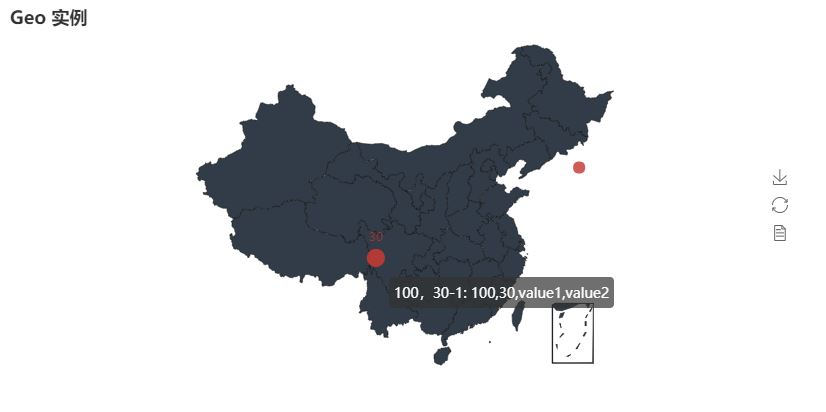﻿ Python 数据可视化pyecharts的使用详解_python_脚本之家
python# Python 数据可视化pyecharts的使用详解

pyecharts 是一个用于生成 Echarts 图表的类库。

echarts是百度开源的一个数据可视化 JS 库，主要用于数据可视化。pyecharts 是一个用于生成 Echarts 图表的类库。实际上就是 Echarts 与 Python 的对接。

pyecharts包含的图表

• Bar（柱状图/条形图）
• Bar3D（3D 柱状图）
• Boxplot（箱形图）
• EffectScatter（带有涟漪特效动画的散点图）
• Funnel（漏斗图）
• Gauge（仪表盘）
• Geo（地理坐标系）
• Graph（关系图）
• HeatMap（热力图）
• Kline（K线图）
• Line（折线/面积图）
• Line3D（3D 折线图）
• Liquid（水球图）
• Map（地图）
• Parallel（平行坐标系）
• Pie（饼图）
• Polar（极坐标系）
• Sankey（桑基图）
• Scatter（散点图）
• Scatter3D（3D 散点图）
• ThemeRiver（主题河流图）
• WordCloud（词云图）

• Grid 类：并行显示多张图
• Overlap 类：结合不同类型图表叠加画在同张图上
• Page 类：同一网页按顺序展示多图
• Timeline 类：提供时间线轮播多张图

pyecharts安装

`pip install pyecharts`

Windows下通过已下的pip命令进行安装

```pip install echarts-countries-pypkg
pip install echarts-china-provinces-pypkg
pip install echarts-china-cities-pypkg
```

Linux下通过以下的pip命令进行安装

```sudo pip3 install echarts-countries-pypkg
sudo pip3 install echarts-china-provinces-pypkg
sudo pip3 install echarts-china-cities-pypkg
sudo pip3 install echarts-countries-pypkg
sudo pip3 install echarts-china-provinces-pypkg
sudo pip3 install echarts-china-cities-pypkg```

• show_config() 打印输出图表的所有配置项
• render() 默认将会在根目录下生成一个 render.html 的文件，支持 path 参数，设置文件保存位置，如 render(r"e:my_first_chart.html")，文件用浏览器打开。

• chart_name = Type() 初始化具体类型图表。
• render() 生成 .html 文件。

Bar（柱状图/条形图）

```from pyecharts import Bar
bar =Bar("我的第一个图表", "这里是副标题")
bar.add("服装", ["衬衫", "羊毛衫", "雪纺衫", "裤子", "高跟鞋", "袜子"], [5, 20, 36, 10, 75, 90])
bar.show_config()
bar.render()``````from pyecharts import Bar
bar =Bar("x 轴和 y 轴交换")
bar.render()``````bar = Bar()
bar.render()```Pie（饼图）

```from pyecharts import Pie
attr =["衬衫", "羊毛衫", "雪纺衫", "裤子", "高跟鞋", "袜子"]
v1 =[11, 12, 13, 10, 10, 10]
pie =Pie("饼图示例")
pie.show_config()
pie.render()```WordCloud（词云图）

```from pyecharts import WordCloud
name =['Sam S Club', 'Macys', 'Amy Schumer', 'Jurassic World', 'Charter Communications', 'Chick Fil A', 'Planet Fitness', 'Pitch Perfect', 'Express', 'Home', 'Johnny Depp', 'Lena Dunham', 'Lewis Hamilton', 'KXAN', 'Mary Ellen Mark', 'Farrah Abraham', 'Rita Ora', 'Serena Williams', 'NCAA baseball tournament', 'Point Break']
value =[10000, 6181, 4386, 4055, 2467, 2244, 1898, 1484, 1112, 965, 847, 582, 555, 550, 462, 366, 360, 282, 273, 265]
wordcloud =WordCloud(width=1300, height=620)
wordcloud.show_config()
wordcloud.render()``````wordcloud =WordCloud(width=1300, height=620)
wordcloud.add("", name, value, word_size_range=[30, 100], shape='diamond')
wordcloud.show_config()
wordcloud.render()```Geo（地理坐标系）

```from pyecharts import Geo
data =[("海门", 9), ("鄂尔多斯", 12), ("招远", 12), ("舟山", 12), ("齐齐哈尔", 14), ("盐城", 15)]
geo =Geo("全国主要城市空气质量", "data from pm2.5", title_color="#fff", title_pos="center", width=1200, height=600, background_color='#404a59')
attr, value =geo.cast(data)
geo.add("", attr, value, type="effectScatter", is_random=True, effect_scale=5)
geo.show_config()
geo.render()```

```#!/usr/bin/env python
# -*- coding:utf-8 -*-
# Author: jyroy
from pyecharts import Geo
if __name__ == '__main__':

list_city_name=["100，30-1","130，40-2"]
list_num = [["value1","value2"],["value3"]]
geo_cities_coords = {"100，30-1":[100,30],"130，40-2":[130,40]}

geo = Geo("Geo 实例")
geo.render()`````` for n, m in data.items(): #data是我从数据库中读取的城市的字典数据
list_1 = []
list_2 = []
list_1.append(n)
list_2.append(m)
try:
geo.add("", list_1, list_2, visual_range=[0, 3000], visual_text_color="#fff", symbol_size=15, is_visualmap=True)
except Exception as e:
pass```Line（折线/面积图）

```from pyecharts import Line
attr =["衬衫", "羊毛衫", "雪纺衫", "裤子", "高跟鞋", "袜子"]
v1 =[5, 20, 36, 10, 10, 100]v2 =[55, 60, 16, 20, 15, 80]
line =Line("折线图示例")
line.add("商家B", attr, v2, is_smooth=True, mark_line=["max", "average"])
line.show_config()
line.render()``````line =Line("折线图-面积图示例")
line.add("商家A", attr, v1, is_fill=True, line_opacity=0.2, area_opacity=0.4, symbol=None)
line.add("商家B", attr, v2, is_fill=True, area_color='#000', area_opacity=0.3, is_smooth=True)
line.show_config()
line.render()```